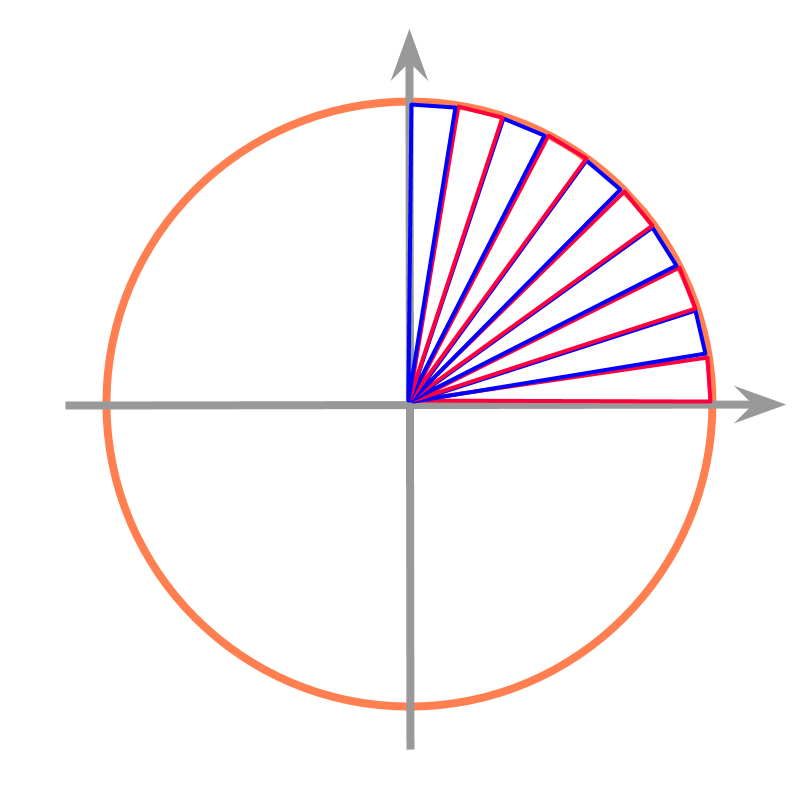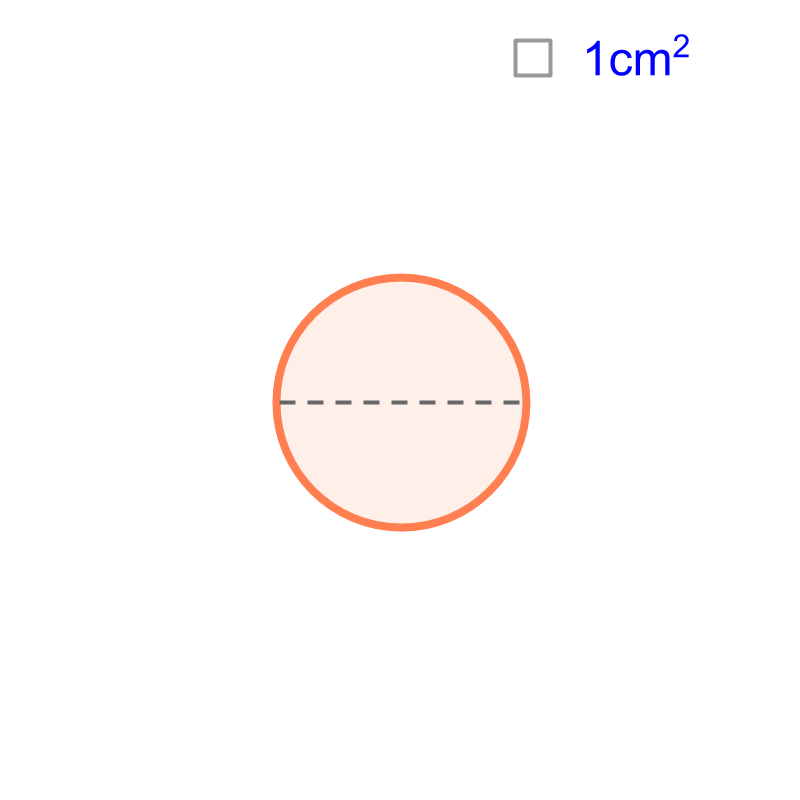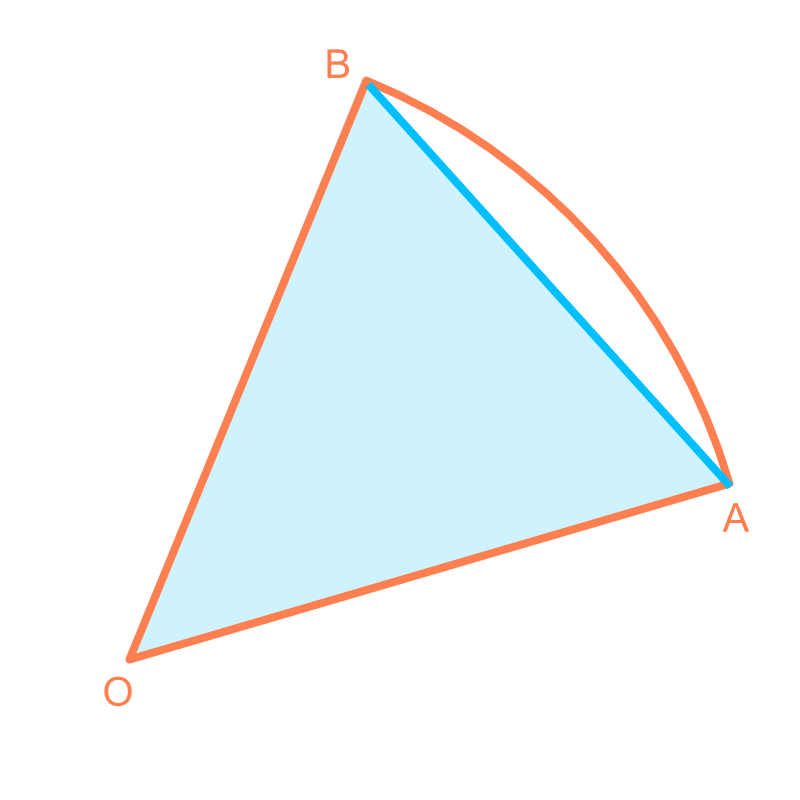maths > mensuration-high

Area of a circle

what you'll learn...

Overview

Area of a Circle : Circumference of a circle of $1$$1$ cm diameter is computed to be a decimal value that does not end or repeat. That accurate value is represented by the symbol $\pi$$\pi$

Area of a circle of $1$$1$ cm diameter is computed to be a decimal value that does not end or repeat. That accurate value is taken to be another value $\alpha$$\alpha$. It can be calculated to be a value by approximating the circle to infinitesimally small triangular pieces.We derive / prove that $\alpha =\pi$$\alpha = \pi$

Area of a circle of $r$$r$ cm radius is computed to be $r$$r$ times the area of the circle of $1$$1$ cm diameter.

Thus the generic formula for the area of a circle is $\pi {r}^{2}$$\pi {r}^{2}$

recap

We have learned the following

•  Length of a real-life object or a line can be a decimal number in which the decimals do not end or do not repeat.

•  Circumference of a circle is $\pi$$\pi$ times the diameter.

Similarly, the area of a circle of $1$$1$ cm radius is also a decimal number in which the decimals do not end or repeat. It is calculated that the area of a circle or radius $r$$r$ is $\pi ×r×r$$\pi \times r \times r$ or $=\pi {r}^{2}$$= \pi {r}^{2}$.

The above was at beginner level understanding. Now, let us learn how to calculate the area of a circle .

calculatingConsider the circle given in the figure. The diameter of the circle is $d$$d$ cm. We visualize the circle as a combination of isosceles-triangles and find sum of the area of triangles as the approximate area of the circle.One of the triangles from the above is isolated and shown along with the corresponding arc of the circle.The area of the triangle $△OAB$$\triangle O A B$ is an approximation to the corresponding sector of the circle $OAB$$O A B$.

To increase the accuracy of the area the triangles are made thinner (angle between equal sides is smaller).

The area of a circle is calculated by one of many methods involving equivalence and approximation.

The area of the circle is the sum of area of the isosceles triangles.

$=$$=$ sum of $\frac{1}{2}×\phantom{\rule{1ex}{0ex}}\text{height}\phantom{\rule{1ex}{0ex}}×\phantom{\rule{1ex}{0ex}}\text{base}$$\frac{1}{2} \times \textrm{h e i g h t} \times \textrm{b a s e}$

$=\frac{1}{2}×\phantom{\rule{1ex}{0ex}}\text{height}\phantom{\rule{1ex}{0ex}}×\left(\phantom{\rule{1ex}{0ex}}\text{sum of bases}\right)$$= \frac{1}{2} \times \textrm{h e i g h t} \times \left(\textrm{\sum o f b a s e s}\right)$

As the triangles are made infinitesimal,

•  the height of the triangle is the radius and

•  the sum of bases is the perimeter So the area is
$=\frac{1}{2}×r×2×\pi ×r$$= \frac{1}{2} \times r \times 2 \times \pi \times r$
$=\pi {r}^{2}$$= \pi {r}^{2}$

summarizing the derivation

Circumference of a circle of $1$$1$ cm diameter is computed to be a decimal value that does not end or repeat. That accurate value is represented by the symbol $\pi$$\pi$

Area of a circle of $1$$1$ cm diameter is computed to be a decimal value that does not end or repeat. That accurate value is taken to be another value $\alpha$$\alpha$

We drived that $\alpha =\pi$$\alpha = \pi$

Area of a circle of $r$$r$ cm radius is computed to be $r$$r$ times the area of the circle of $1$$1$ cm diameter.

Thus the generic formula for the area of a circle is $\pi {r}^{2}$$\pi {r}^{2}$

What is the area of a circle of $\frac{1}{\pi }$$\frac{1}{\pi}$ cm radius?
The answer is "$\frac{1}{\pi }c{m}^{2}$$\frac{1}{\pi} c {m}^{2}$".

Area

$=\pi {r}^{2}$$= \pi {r}^{2}$

$=\pi ×\frac{1}{\pi }×\frac{1}{\pi }$$= \pi \times \frac{1}{\pi} \times \frac{1}{\pi}$

$=\frac{1}{\pi }c{m}^{2}$$= \frac{1}{\pi} c {m}^{2}$

summary

Area of a Circle :
Circumference of a circle of $1$$1$ cm diameter is computed to be a decimal value that does not end or repeat. That accurate value is represented by the symbol $\pi$$\pi$

Area of a circle of $1$$1$ cm diameter is computed to be a decimal value that does not end or repeat. That accurate value is taken to be another value $\alpha$$\alpha$. It can be calculated to be a value by approximating the circle to infinitesimally small triangular pieces.We derive / prove that $\alpha =\pi$$\alpha = \pi$

Area of a circle of $r$$r$ cm radius is computed to be $r$$r$ times the area of the circle of $1$$1$ cm diameter.

Thus the generic formula for the area of a circle is $\pi {r}^{2}$$\pi {r}^{2}$

Outline